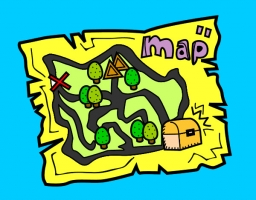# Triangle-shaped 30011

Determine the map's scale if the 1.6 km, 2.4 km, and 2.7 km triangle-shaped forests are drawn on the map as a triangle with sides of 32 mm, 48 mm, and 54 mm.

M =  50000

### Step-by-step explanation:Did you find an error or inaccuracy? Feel free to write us. Thank you!

Tips for related online calculators
Need help calculating sum, simplifying, or multiplying fractions? Try our fraction calculator.
Check out our ratio calculator.
Do you want to convert length units?

#### Grade of the word problem:

We encourage you to watch this tutorial video on this math problem: